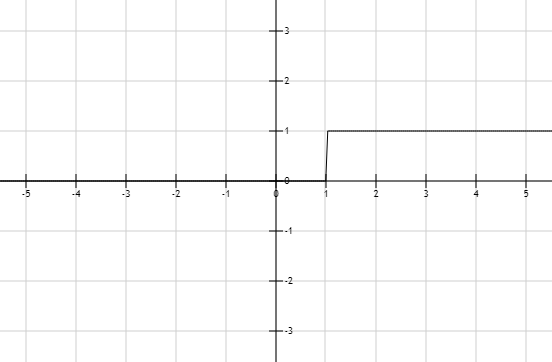# The solution of given linear inequality equation and plot a graph for it.### Precalculus: Mathematics for Calcu...

6th Edition
Stewart + 5 others
Publisher: Cengage Learning
ISBN: 9780840068071### Precalculus: Mathematics for Calcu...

6th Edition
Stewart + 5 others
Publisher: Cengage Learning
ISBN: 9780840068071

#### Solutions

Chapter 1.7, Problem 19E
To determine

## The solution of given linear inequality equation and plot a graph for it.

Expert Solution

x1

### Explanation of Solution

Given:

Linear inequality equation

3x+116x+8

Calculation,

Linear inequality equation,

3x+116x+83x6x8113x33x3x33x1

Now, graph of 3x+116x+8Conclusion:

Hence, the values of x is less than equal to the 1.

### Have a homework question?

Subscribe to bartleby learn! Ask subject matter experts 30 homework questions each month. Plus, you’ll have access to millions of step-by-step textbook answers!